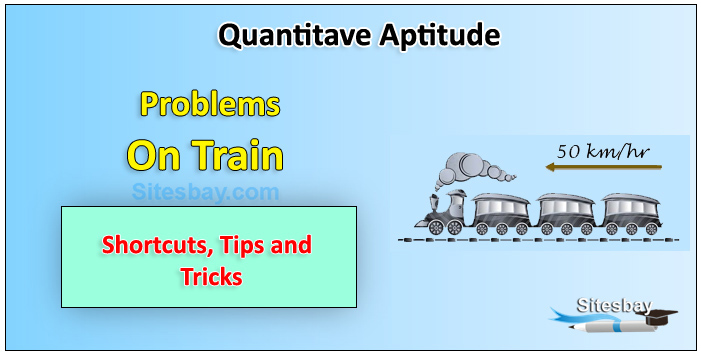# Train Problem

## Aptitude on Train Problem

Speed: Speed is defined as the distance covered by an object in unit time.### Importation Rules for Train Problem

• When two trains are moving in opposite directions, then relative speed will be the addition of their individual speeds.
• When two trains are moving in same direction, then relative speed will be the subtraction of their individual speeds.
• When a train passes through a pole or person standing, net distance traveled to pass is the length of the train
• On passing a platform by a certain train the net distance traveled is the sum of length of train and the length of platform both.

### Two Train Moving in Opposite Direction Problem

When two trains are moving in opposite directions, then relative speed will be the addition of their individual speeds.

Example Two trains 125 m and 100 m in length respectively are running in opposite directions, one at the rate of 50 km / hr and the other at the rate of 40 km /hr. At what time they will clear each other from the moment they meet ?

## Solution

```Relative speed of trains = (50 + 40) km/hr = [90 * ( 5 / 18 )] = 25 m/sec

Total length to be traveled = 125 + 100 = 225 m

Time Taken = (225/25) = 9 sec
```

Example A train 125 m in length, moves at a speed of 82 km / hr , In what time the train will cross a boy who is walking at 8 km / hr in opposite direction ?

## Solution

```Relative speed = 82+8 = 90 km / hr = [ 90 * ( 5 / 18 )] = 25 m / sec

Time Taken = (125/25) = 5 sec
```

### Train and Pole or Man Problem

When a train passes through a pole or person standing, net distance traveled to pass is the length of the train.

Example A train 120 m long is running at the speed of 54 km /hr. Find the time taken it to pass a man standing near the railway track.

## Solution

```speed of train = [54 * ( 5 / 18 ) ] = 15 m / sec

length of train = 120 m , So required time:

Time taken = (120/15) = 8 sec
```

### Train and Platform Rule

On passing a platform by a certain train the net distance traveled is the sum of length of train and the length of platform both.

Example A train is moving at a speed of 54 km / hr. If the length of the train is 100 meters, how long will it take to cross a railway platform 110 meters long ?

## Solution

```speed of train = [54 * ( 5 / 18 ) ] = 15 m / sec

Distance covered in passing the platform = 100 + 110 = 210 m

Time Taken = (210/15) = 14 sec
```

### Two Train moving in Same Direction Problem

When two trains are moving in same direction, then relative speed will be the subtraction of their individual speeds.

Example Two trains 110 m and 100 m in length respectively are running in same directions, one at the rate of 100 km / hr and the other at the rate of 64 km / hr. At what time faster train will clear other train from the moment they meet ?

## Solution

```Since trains are running in same direction,
so relative speed = 100-64 = 36 km/hr = [ 36 * ( 5 / 18 )] = 10 m/sec

Total length to be traveled = 110 + 100 = 210 m

Time Taken = (210/10) = 21 sec
```

Example A train passes a standing pole on the platform in 5 seconds and passes the platform completely in 20 seconds. If the length of the platform is 225 meters. Then find the length of the train ?

## Solution

``` Let the length of the train is x meter

So speed of train =( x / 5 ) m / sec

Also speed of train = ( 225 + x ) / 20   m/sec

x/5 = 225+x/20

x=75m
```

Example Two trains of length 115 m and 110 m respectively run on parallel rails. When running in the same direction the faster train passes the slower one in 25 seconds, but when they are running in opposite directions with the same speeds as earlier, they pass each other in 5 seconds. Find the speed of each train ?

## Solution

```Let the speed of trains be x m/sec and y m/sec e-respectively.

When they move in same direction their relative speed is : x - y

When they moves in opposite direction their relative speed is : x + y

x-y = (115+110)/25

x+y = (115+110)/5

On solving two equations x=27 m/s and y=18 m/sec
```